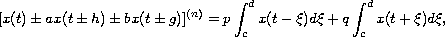Electron. J. Diff. Equ., Vol. 2010(2010), No. 23, pp. 1-10.

### Oscillation of solutions for odd-order neutral functional differential equations Tuncay Candan

Abstract:
In this article, we establish oscillation criteria for all solutions to the neutral differential equationswhereis odd,,,andare nonnegative constants. We consider 10 of the 16 possible combinations of +/- signs, and give some examples to illustrate our results.

Submitted December 9, 2009. Published February 4, 2010.
Math Subject Classifications: 34K11, 34K40.
Key Words: Neutral differential equations; oscillation of solutions; distributed deviating arguments.

Show me the PDF file (204 KB), TEX file, and other files for this article.Tuncay Candan Department of Mathematics, Faculty of Art and Science Nigde University, Nigde, 51200, Turkey email: tcandan@nigde.edu.tr